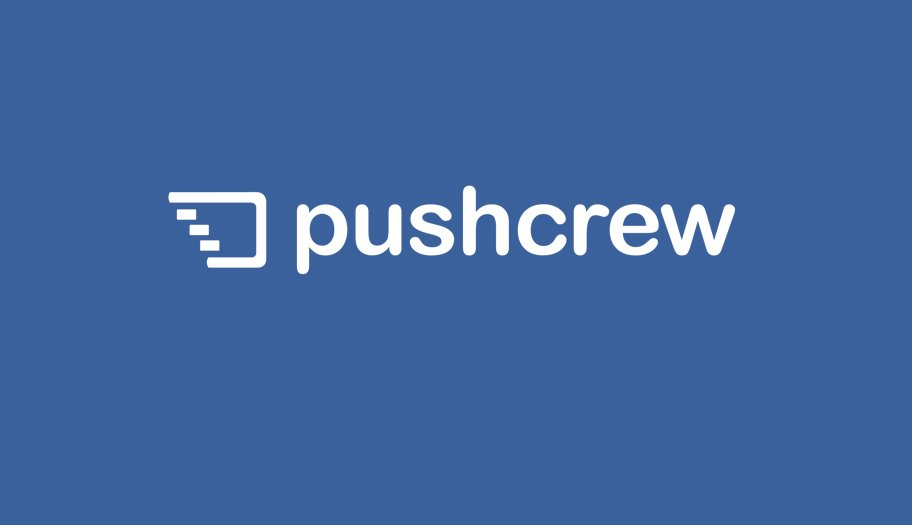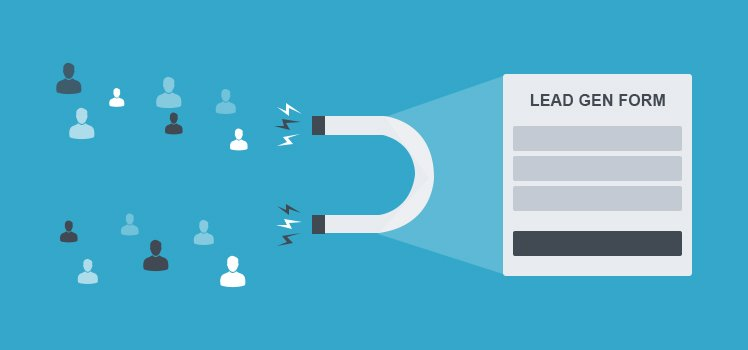We tweet useful stuff daily

# How to benchmark competition conversion rates using Alexa in two super simple stepsLet’s face the truth; Alexa is not the best source of traffic data out there on the Internet. Plus, it does not have statistics on conversion rates. But, hey, Alexa is free and we are going to use it to benchmark (approximately) the conversion rates for your competition. Here is how to do it two simple steps:

Step 1. Establish industry norms using your actual conversion rate data

Suppose you are SEOMoz (I am using this website as an example and have no real data for them) and you sell paid tools for SEO. Let’s suppose your current conversion rate is a conservative 4% (again, hypothetical data) and you want to estimate how your competitor SEO Book is doing.

You and your competitors (since it is the same industry after all) follow a similar trend when it comes to relationship between conversion rates and other site metrics such as bounce rate, time on site, and page views per user. In this step, we try to calculate values for parameters which relate conversion rate to all these metrics. Using your actual conversion rate data and the stats that Alexa shows about your website, calculate X, Y and Z as the following:

• Your Conversion Rate = X * (1 / Bounce Rate as shown by Alexa )
• Your Conversion Rate = Y * Time Spent on Site as shown by Alexa (in seconds)
• Your Conversion Rate = Z * Page views / user as shown by Alexa

The reason we don’t use your actual bounce rate, time spent and page views data is because you don’t have that data for your competitors. You only know what Alexa says about your website and what Alexa says about your competitors. So it is better to work on the Alexa data that is freely available and uses the same methodology all across.

As an example of SEOMoz, Alexa tells the bounce rate, time spent on site and page views / user is 50.7%, 219.7 seconds and 3.2 respectively. Using this data, we get the values of X, Y and Z as:

• X = 4% / (1 / 50.7 %) = 201.6
• Y = 4% / 219.7 = 0.018
• Z = 4% / 3.2 = 1.25

Step 2.  Use the parameters to estimate competitor’s conversion rate

Now we have obtained the parameters which relate your actual conversion rate to the data that Alexa shows about your website. Next step is to simply use those parameters on your competitor’s data (as shown by Alexa) to get estimates of their conversion rate.

Competitor Conversion Rate:

• Estimate 1 = X * (1 / Competitor Bounce Rate as shown by Alexa)
• Estimate 2 = Y * Competitor Time spent on Site as shown by Alexa
• Estimate 3 = Z * Competitor Page views / user as shown by Alexa

Finally, to get an idea of what their real conversion rate, we simply average the estimates.

Competitor Conversion Rate = (Estimate 1 + Estimate 2 + Estimate 3) / 3

Continuing the SEOMoz example, if we were to estimate the conversion rates for SEOBook, we calculate 3 estimates of conversion rate (based on the data shown by Alexa for SEOBook):

• Estimate 1 = 201.6 * (1/69.4) = 2.90%
• Estimate 2 = 0.018 * 135 = 2.43%
• Estimate 3 = 1.25 * 2.43 = 3.0%

As you can observe, the estimates are quite close. Hence, we can be pretty confident that the actual conversion rate is close to the average of these three estimates, which is:

Estimated conversion rate = 2.77%

Of course, the above estimated value is only valid if the real conversion rate for SEOMoz that I assumed (4%) is true, which may or may not be the case as I don’t have access to their real web analytic data.

Summary

Simply plug in your conversion rates in the above methodology and you should have pretty good estimate on how you are doing as compared to your competitors. You can also try to triangulate your estimates by using other data sources (apart from Alexa) such as Compete.com or Quantcast.

Do let me know if you find this approach helpful.  As always, feedback and comments appreciated. Want a tool to automate all this analysis for you?

PS: The way I define Bounce Rate and Conversion Rate, they are not related.  But the way Alexa defines, the two metrics are definitely related.Paras Chopra Founder and Chairman of VWO
##### More from VWO on Conversion Rate Optimization## Sorry, We Messed Up

It has come to our notice that a new beta feature that we switched on…Paras Chopra## What You Should Know About the Optimizely “Vulnerability”

Update 25th June 2014 7pm IST: With the new version of VWO, we have now…Paras Chopra## 9 Actionable Tips to Build An Effective Lead Magnet

Converting website visitors into customers — as we all know — is not simple. It’s…Nitin Deshdeep